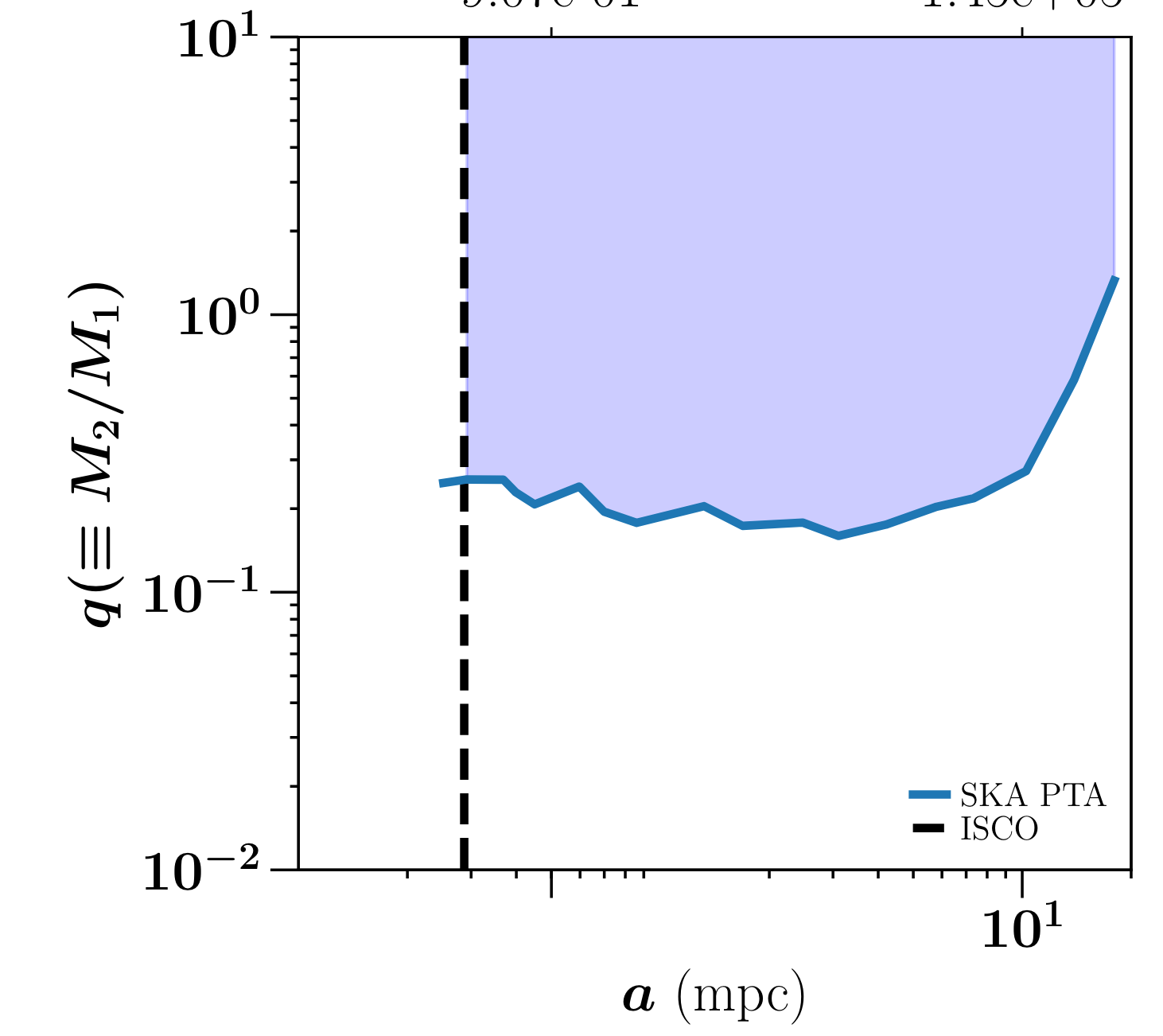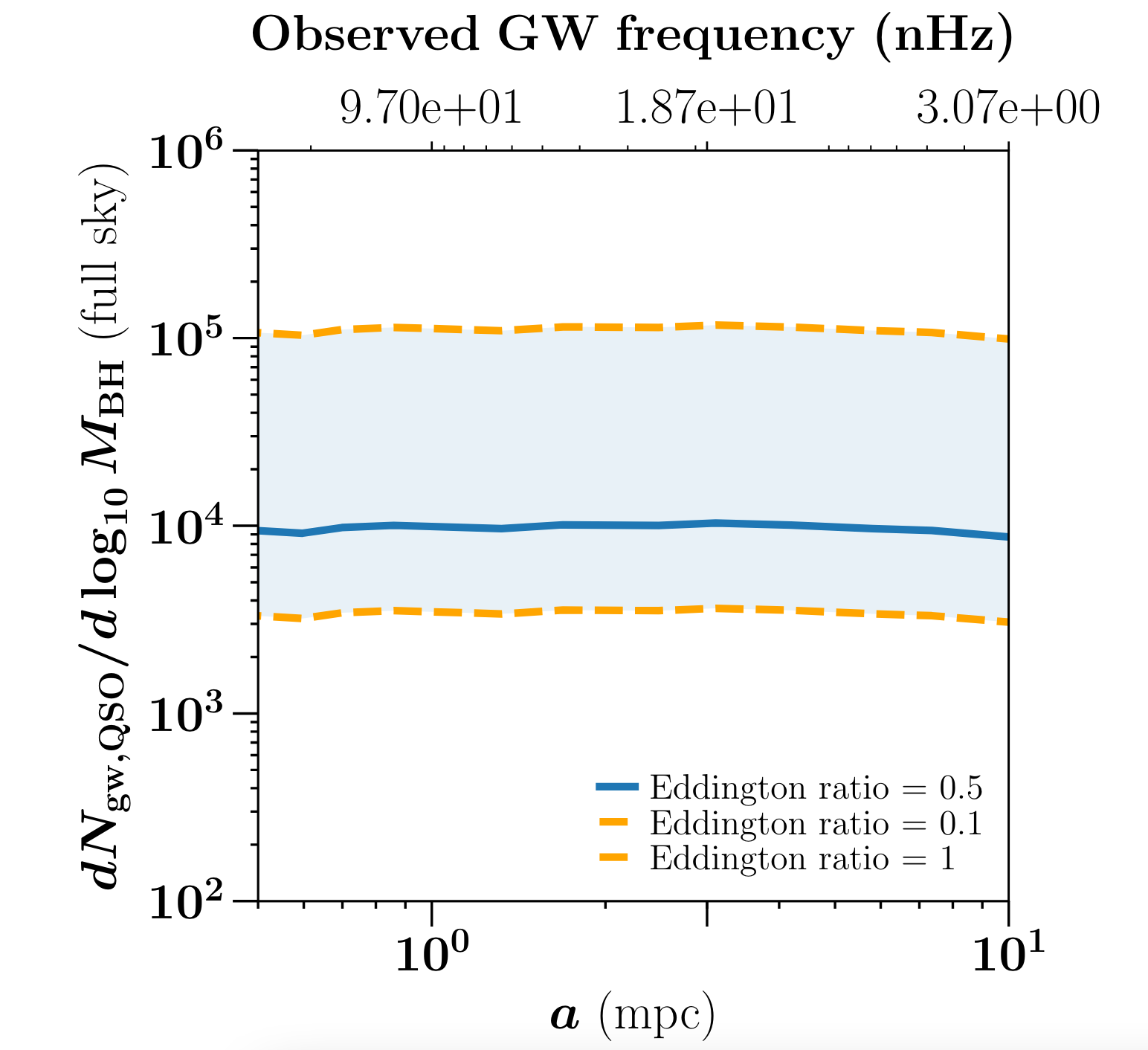# Pulling Back the Curtain on the Formation of the First Supermassive Black Holes

First Author’s Institution: Département de Physique Théorique, Université de Genève, Genève, Switzerland

Status:  open access on the arXiv

In recent years, observations from collaborations such as the Event Horizon Telescope (EHT) and LIGO (and many others) and advancements in numerical simulations (e.g., this Astrobite) have revolutionized our understanding of black holes (BHs) in the local universe. However, the formation and growth of the first supermassive black holes remains an open question in the field. Our observations of some active black holes known as quasars at high redshifts show that they are able to grow to hundreds of millions of times the mass of our Sun in the first billion years of the universe. While this timescale may seem long, cosmologically speaking, it is actually quite short! This sets a fundamental – and yet unsettled – question in the field: how did they get so big so quickly?

In our current understanding of the universe, structures form hierarchically, meaning the smallest structures collapse first, then iteratively merge to form larger and larger structures until we get the distribution of matter that we see in the universe today. As a result of this, mergers of galaxies hosting supermassive BHs (SMBHs) in their centers are believed to give rise to systems of binary SMBHs which will themselves eventually merge. This, in part, is believed to drive the growth of SMBHs in the early universe.

Therefore, if we can observe the signatures of these mergers – such as gravitational waves (GWs) and potential electromagnetic counterparts – we can constrain our models describing the growth of these young SMBHs. However, rather than probing the GW signatures directly (as is done with instruments such as LIGO), the focus of today’s paper is on using the Square Kilometre Array (SKA) PTA to measure the background of GWs across the sky. I know what you may be thinking, but no, the SKA does not have a parent-teacher association put in charge of measuring the all-sky gravitational wave background – this PTA stands for pulsar timing array. This astrobite provides an excellent overview of pulsar timing arrays, which rely on the precise, periodic pulses we observe from rapidly rotating neutron stars (known as pulsars). When a pulse passes through a background of low-frequency (nanohertz range) gravitational waves, it arrives at a slightly different time than we expect, so measuring such deviations serves as a proxy for observing these GWs. With this method, we measure the GW background – or the collective effects of a large number of GW sources randomly emitting GWs and modifying pulse times – and can statistically disentangle individual GW events from the combined signal. As a result, PTAs are a powerful tool with which we can study the low-frequency events which are drowned out for ground-based interferometers such as LIGO.

With the SKA PTA in mind, today’s authors quantify the detectability of SMBH binary mergers and describe how these measurements can reinforce our models of SMBH growth.

How low can you go?

The first question that today’s paper tackles is what population of SMBH binaries the SKA PTA will be able to detect. By combining the frequency limits and GW amplitude (or, more precisely, strain) that are detectable by SKA, the authors calculate the range of binary separations and mass ratios that the instrument should be able to detect (see Figure 1). In doing so, they demonstrate that the detectable mass ratios are relatively independent of binary separation (note how the lines flatten to the left of Figure 1) and that SKA won’t be able to detect binaries for which the more massive progenitor is less than a trillion solar masses.Figure 1: The detection threshold of the SKA PTA in terms of the binary separation (a) and the mass ratio of the two progenitor black holes (q). The shaded region represents the detectable region of black hole mergers and the vertical dashed line indicates the innermost stable circular orbit (ISCO) of the more massive progenitor black hole. This threshold is computed for a primary (more massive) progenitor with a mass of a trillion solar masses. (adapted from Figure 5 in the paper)

Next, they want to estimate the number of BH binaries that should be detectable at a given time. To do this, they use the merger rate of dark matter (DM) halos of galaxies in conjunction with the empirical relationship between DM halo and BH mass to get an estimate of the number density of binary BH mergers for a given mass ratio and redshift interval. By integrating this over the range of observable mass ratios (from Figure 1) and multiplying by the volume of the region at a given redshift, they have an estimate for the number of mergers we’d expect to see with SKA.

From whence did they come?

The next step is to see if we can figure out where the GW event happened. The SKA telescopes are still in development, but optimistic models expect that the telescope will be able to localize sources to a region of 70 deg2. This can be used in tandem with expectations for forthcoming survey telescopes such as the Rubin Observatory to put that number in context: there are on the order of 100,000 galaxies in the SKA localization region at a redshift of 6.8 that might host the black holes of interest. Evidently, electromagnetic signals from quasar counterparts will be necessary to uniquely identify the source of these signals.

Using the quasar luminosity/mass function – which describes the number density of quasars in a luminosity/mass interval – and the mass function of all black holes (both active and not), the authors can compute the “active fraction” of black holes (the ratio of these two mass functions). Given that they already computed the number of binary mergers they expect to see, multiplying this by the fraction of black holes that are active (read: are quasars) gives the number of detectable GW events that are coming from a quasar source (Figure 2). In fact, this calculation helps probe an important physical quantity related to the growth of BHs: the Eddington ratio. This is the ratio between the observed luminosity of the quasar and its Eddington luminosity (the maximum luminosity it can have without the radiation pressure overcoming its gravitational force and breaking it apart) and helps characterize the flow of material onto the BH as it grows. Figure 2 shows the calculation of the number of detectable quasar-sourced GW events for various values of the Eddington ratio and demonstrates that it significantly affects the number of events we might expect.Figure 2: The number of detectable GW events associated with a quasar progenitor across the whole sky for various values of the Eddington ratio and as a function of the separation of the binary (a). Note how varying the Eddington ratio significantly changes the number of detectable events. (adapted from Figure 8 in the paper)

Noting that this calculation is for the entire sky of quasars, they then limit this calculation to the aforementioned localization region of the SKA PTA, finding that there should be a handful (tens) of active quasar counterparts to mergers with a trillion solar mass BH as the more massive progenitor. This indicates that the SKA PTA has great potential to be able to help uniquely identify the electromagnetic counterparts to the most massive black holes in this time in the early universe, hopefully shedding light on the growth of these dark objects.

Astrobite edited by Olivia Cooper

Featured image credit: adapted from NASA Goddard Space Flight Center